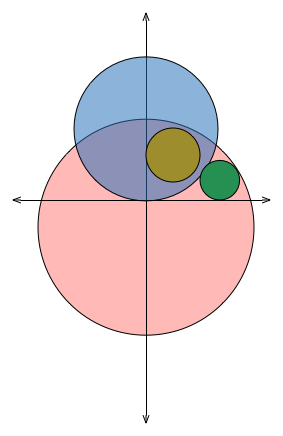# Pushing the boundaries

Geometry Level 5Let circle $A$ have the equation $x^{2} + (y - 2)^{2} = 4$ and circle $B$ have the equation $x^{2} + (y + 1)^{2} = 9$.

Now let $C_{1}$ be the circle in the first quadrant that is tangent to $A, B$ and the $y$-axis, and $C_{2}$ be the circle in the first quadrant that is tangent to $A, B$ and the $x$-axis.

If the center of $C_{1}$ is at $(x_{1}, y_{1})$ and the center of $C_{2}$ is at $(x_{2}, y_{2})$, then find

$(\dfrac{x_{2}}{y_{2}})*(x_{1} + 3y_{1})$.

Clarification: To guarantee a unique solution, both $C_{1}$ and $C_{2}$ must lie inside $B$ and must both lie entirely within the first quadrant, (inclusive of the $x$ and $y$ axes).

×